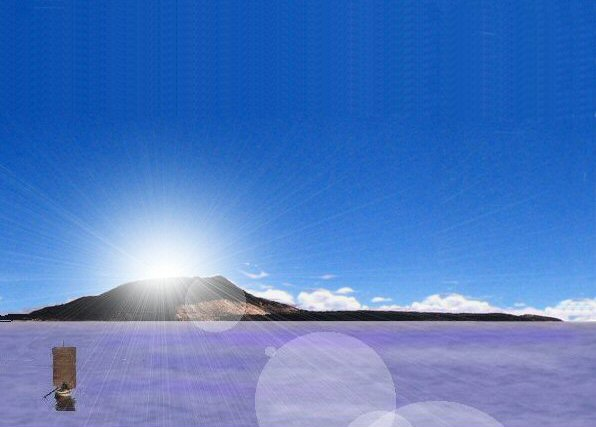### Monte Alban cubits and measurement units.

This essay follows on from earlier studies including Teotihuacan and Chichen Itza to determine the measuring units used in ancient Meso America.

It was found in earlier studies that a "Sumerian" foot of 13.2" was used in the above sites, in conjunction with "Sumerian" cubits of 19.8", "Sumerian" yards of 33.0" which also related to "Egyptian" royal cubits of 20.625" and "Mayan hunabs" which were 2 x Egyptian royal cubits.

All the above measuring units belonged originally to the same measuring system where a double Sumerian yard was 100 Sumerian shusi with Sumerian yard being 50 shusi, Sumerian cubit 30 shusi and Sumerian foot 20 shusi etc.

The Sumerian cubit was 24/25 of the Egyptian royal cubit and may have had its origins in a plot of land which measured 100 Sumerian cubits giving a corresponding distance of 96 Egyptian Royal cubits of 20.625" or 48 Mayan hunabs of 41.25", the differences being whether you preferred to divide by halves, quarters or thirds etc.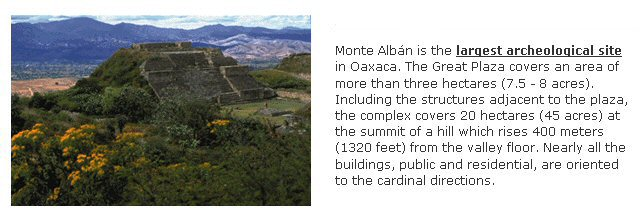The first thing that catches one's attention is that it was built at a height of 1320 feet above the valley floor. See http://www.oaxacaoaxaca.com/monte-alban.htm

That is remarkable because 1320 ft is 1200 Sumerian feet, or 800 Sumerian cubits, or 480 Sumerian yards or 240 Sumerian double yards of 100 shusi, but in "Mayan hunabs" it would come to what seems at first an odd number i.e. 384 hunabs, but as pointed out by Terence Hogan, 384 is 16 x 15ths of the favoured number, 360. And it appears that 1320 ft or 1200 Sumerian feet is no random number, because the mountain top was artificially levelled to build the city.

More detailed information is given on the webpage of Michael Blaschke
"The ancient Zapotec capital Monte Albán, located spectacularly 10 km (6 miles) west of Oaxaca city on an artificially levelled mountain top, is the second largest ceremonial site in Mesoamerica, only exceeded in size by Teotihuacán near Mexico City.

Monte Albán I: Starting from the first occupation about 500 BC, up to about 200 BC, most probably by the Zapotecs, although it likely had early cultural connections with the Olmecs to the northeast. The top of the mountain, a stubborn quartzite, was hewn off with stone chisels until a flat area was formed 3,117 feet long and 1,476 feet wide, and the debris was used to fill crevasses on the edges of the platform. Temples and probably palaces were built, and a town of 10,000 or more people grew on the hillsides."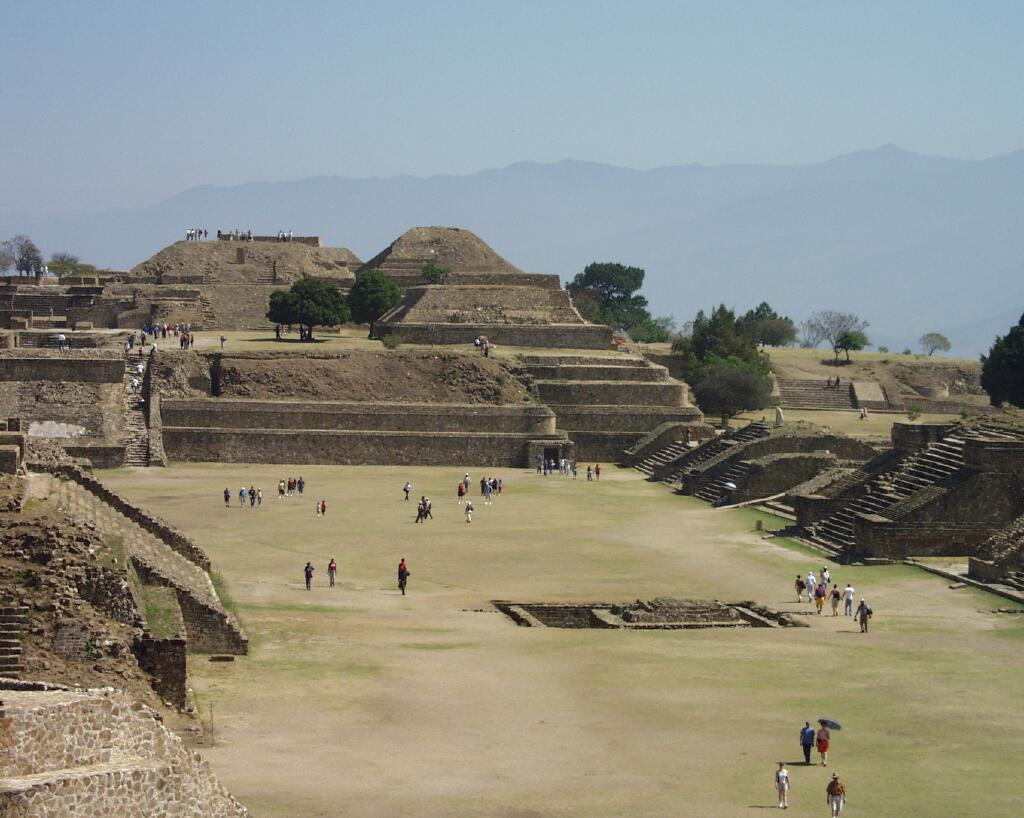East side of Gran Plaza and North Platform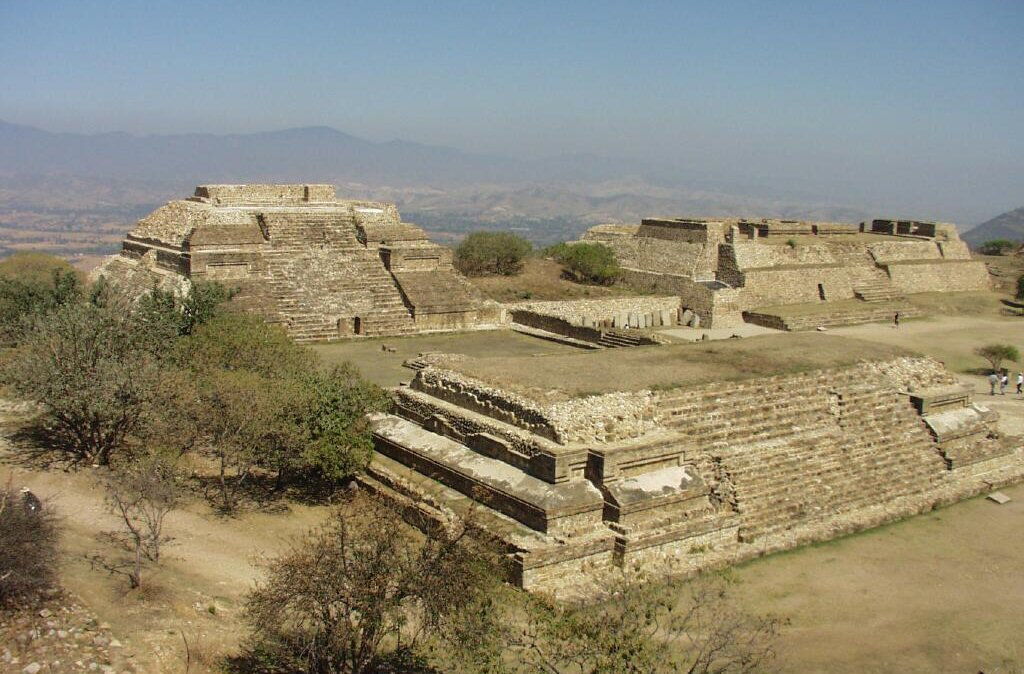buildings known as sistema L, danzantes and M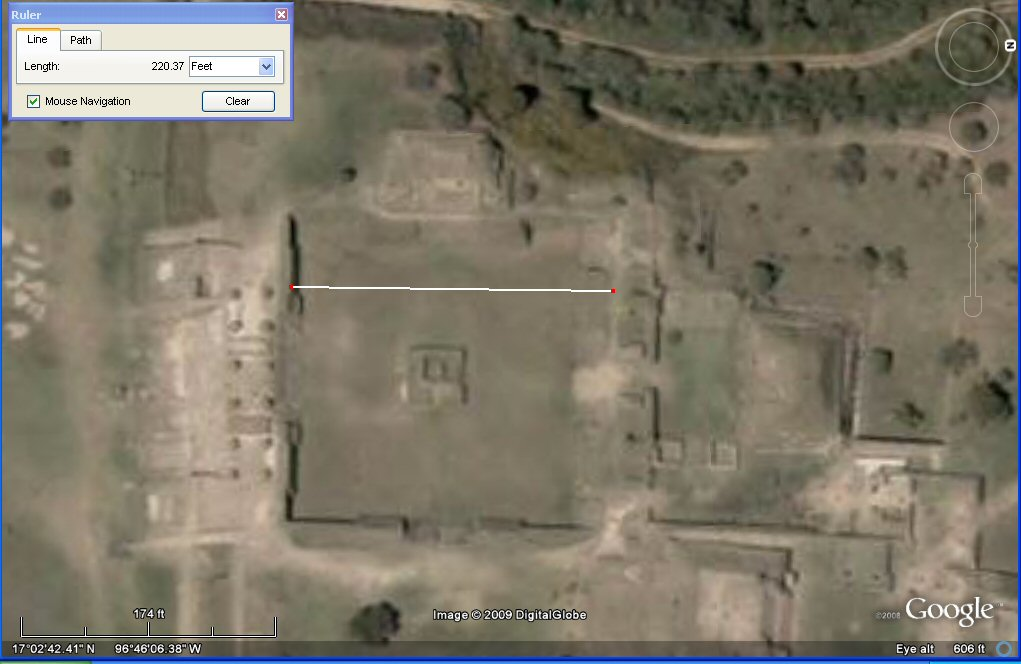The satellite measures the sunken plaza of the North platform as 220 ft which would be 200 Sumerian feet.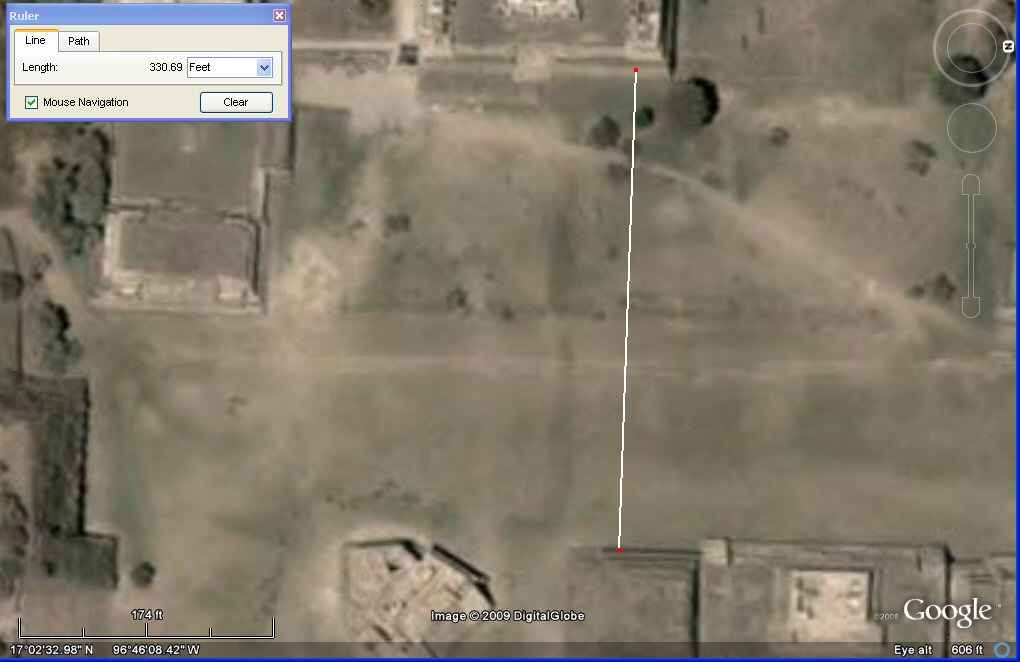This part of the gran plaza measures 330ft which is 300 Sumerian ft or 200 Sumerian cubits wide
or 2 x stades of 165ft (150 Sumerian ft)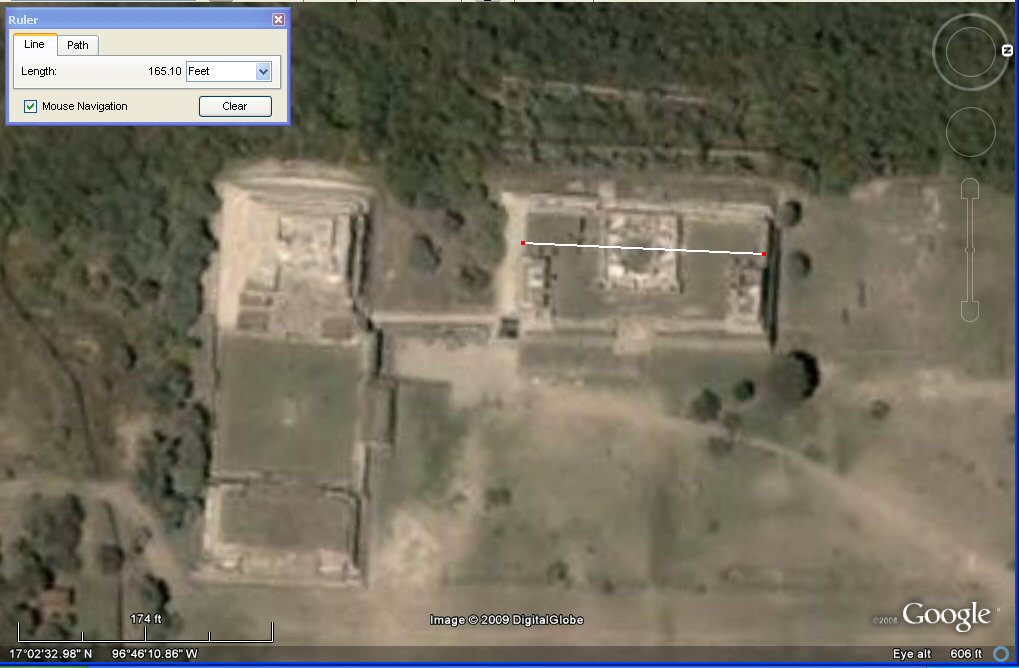Edifice L measures 165ft which is 150 Sumerian feet or 100 Sumerian cubits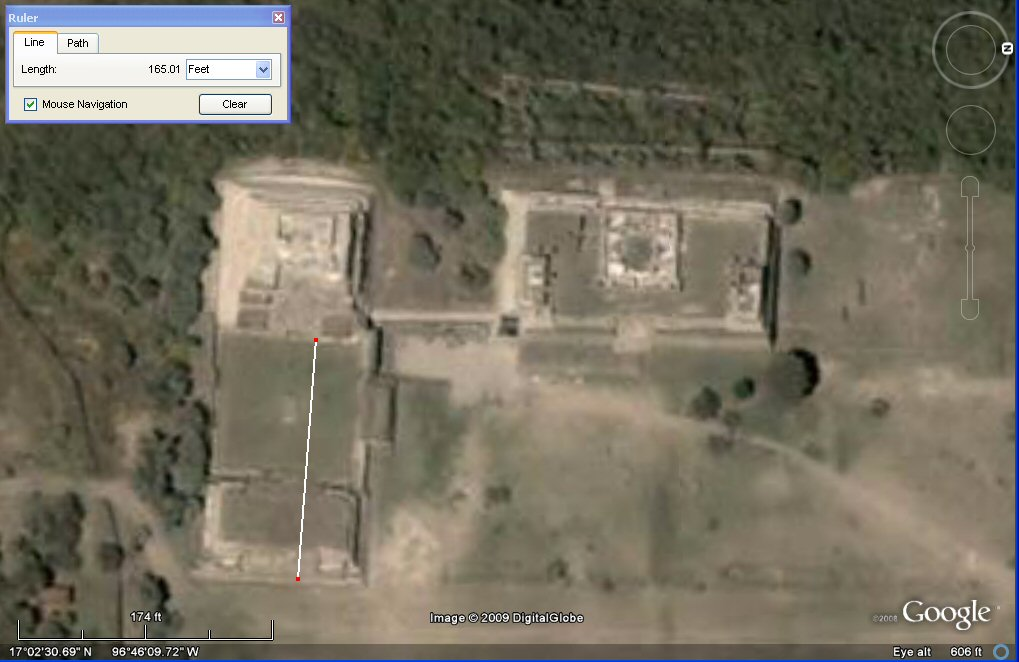Edifice M measures 165ft which is 150 Sumerian feet or 100 Sumerian cubits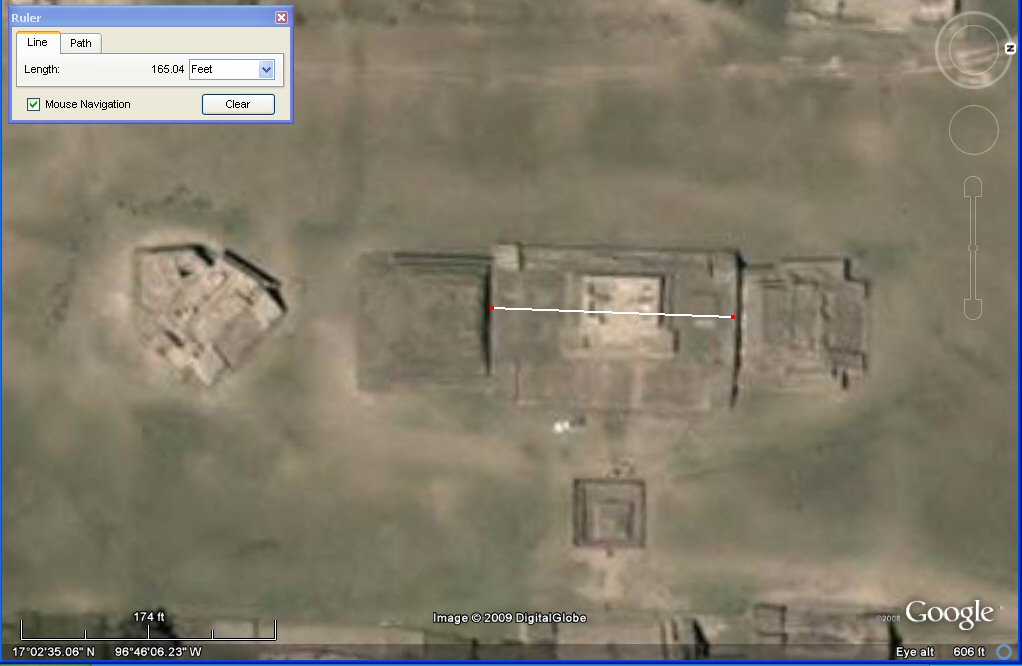Edifice H measures 165ft which is 150 Sumerian feet or 100 Sumerian cubits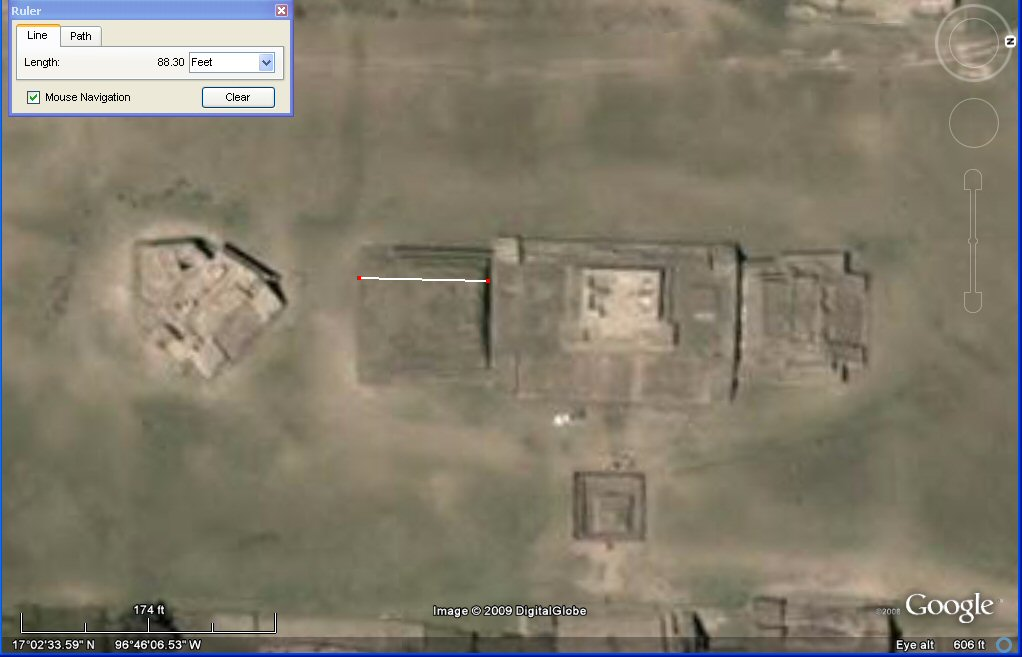Edifice I measures 88 ft which is 80 Sumerian feet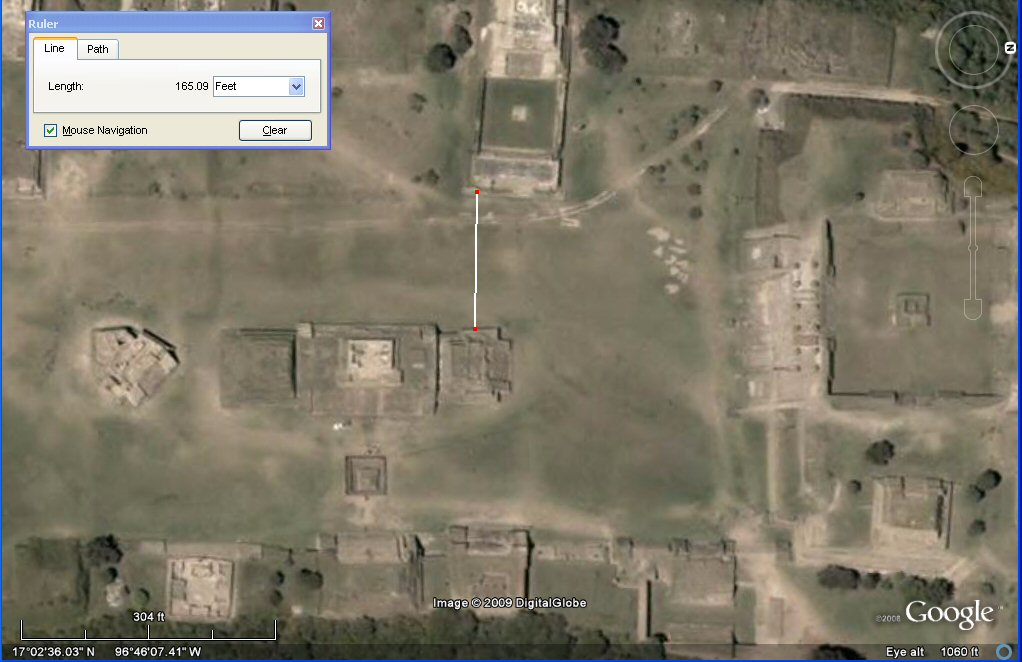This part of the plaza measures 165ft which is 150 Sumerian feet or 100 Sumerian cubits wide.

Although the satellite images may not be as accurate as measurements on the ground, at the same time many of these ancient sites have been "reconstructed" and we don't know if they were set out with any particular degree of accuracy to begin with.

However, the measurements enable us to reconstruct a system of measurement which seems to have become fractured and dissipated throughout the world with Mexico remaining one of the places where all the various aspects of this system can still be detected.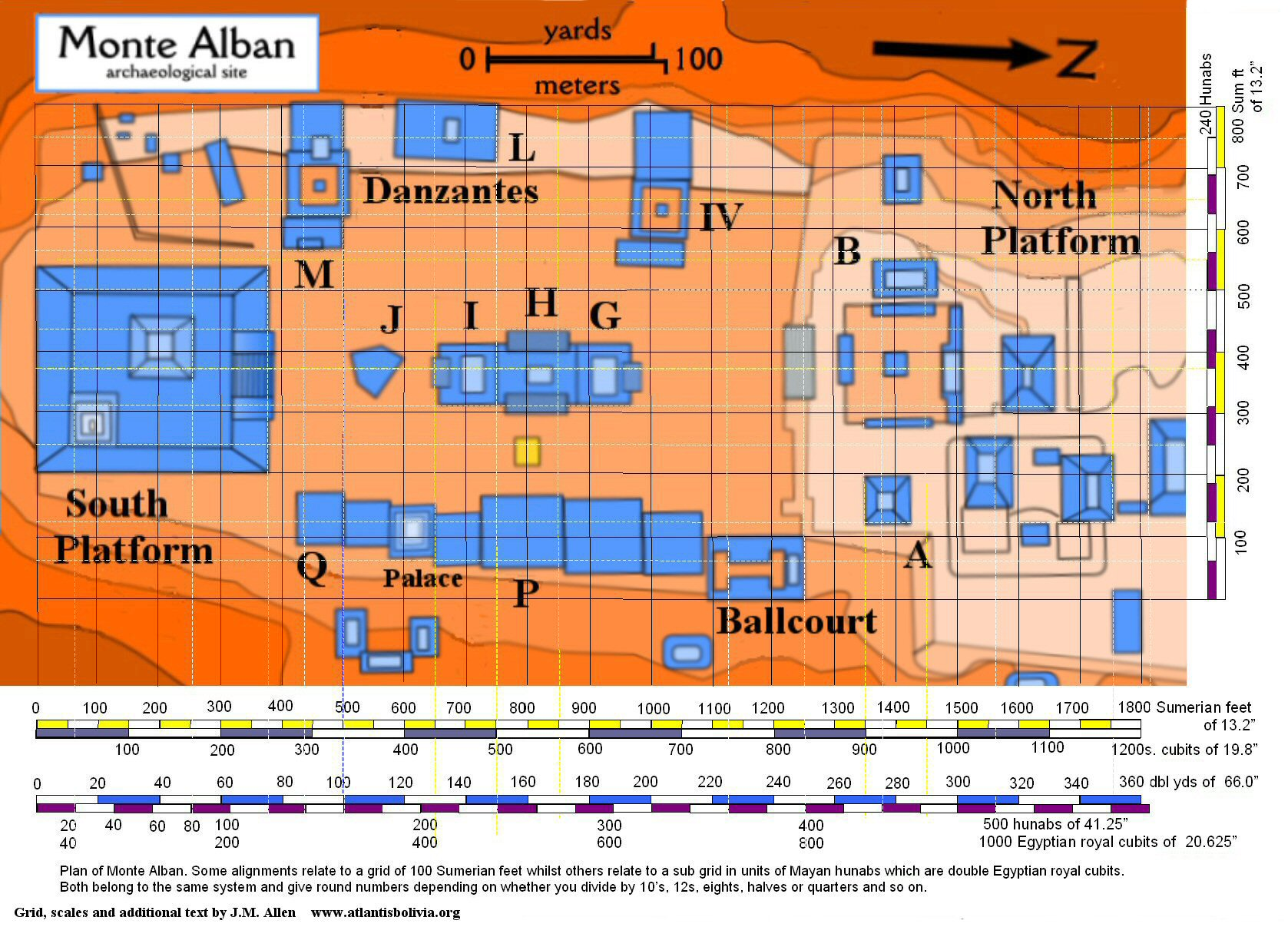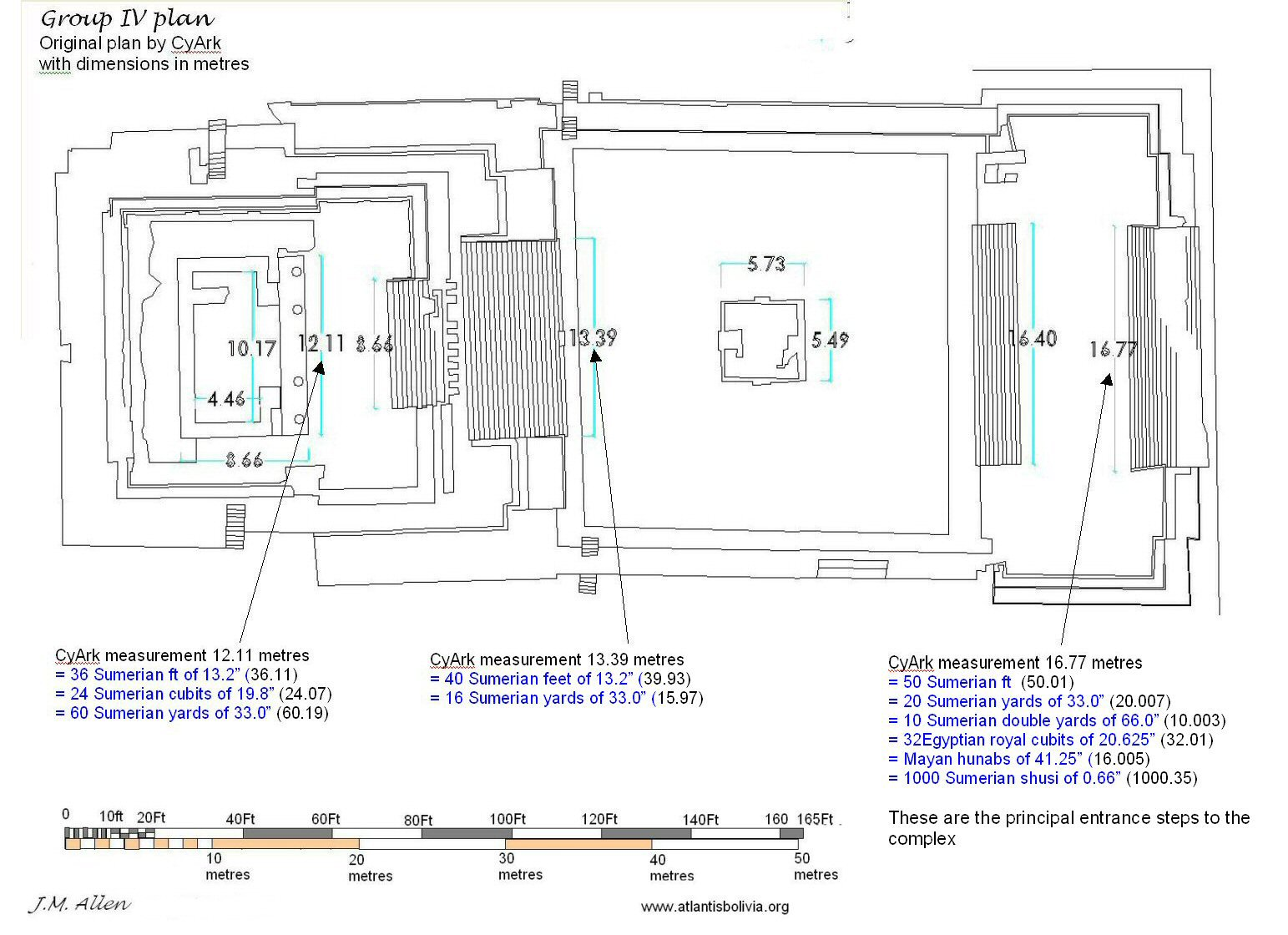above, the main steps to the outer complex measure 50 Sumerian feet of 13.2"
and the steps to the inner complex measure 40 Sumerian feet of 13.2".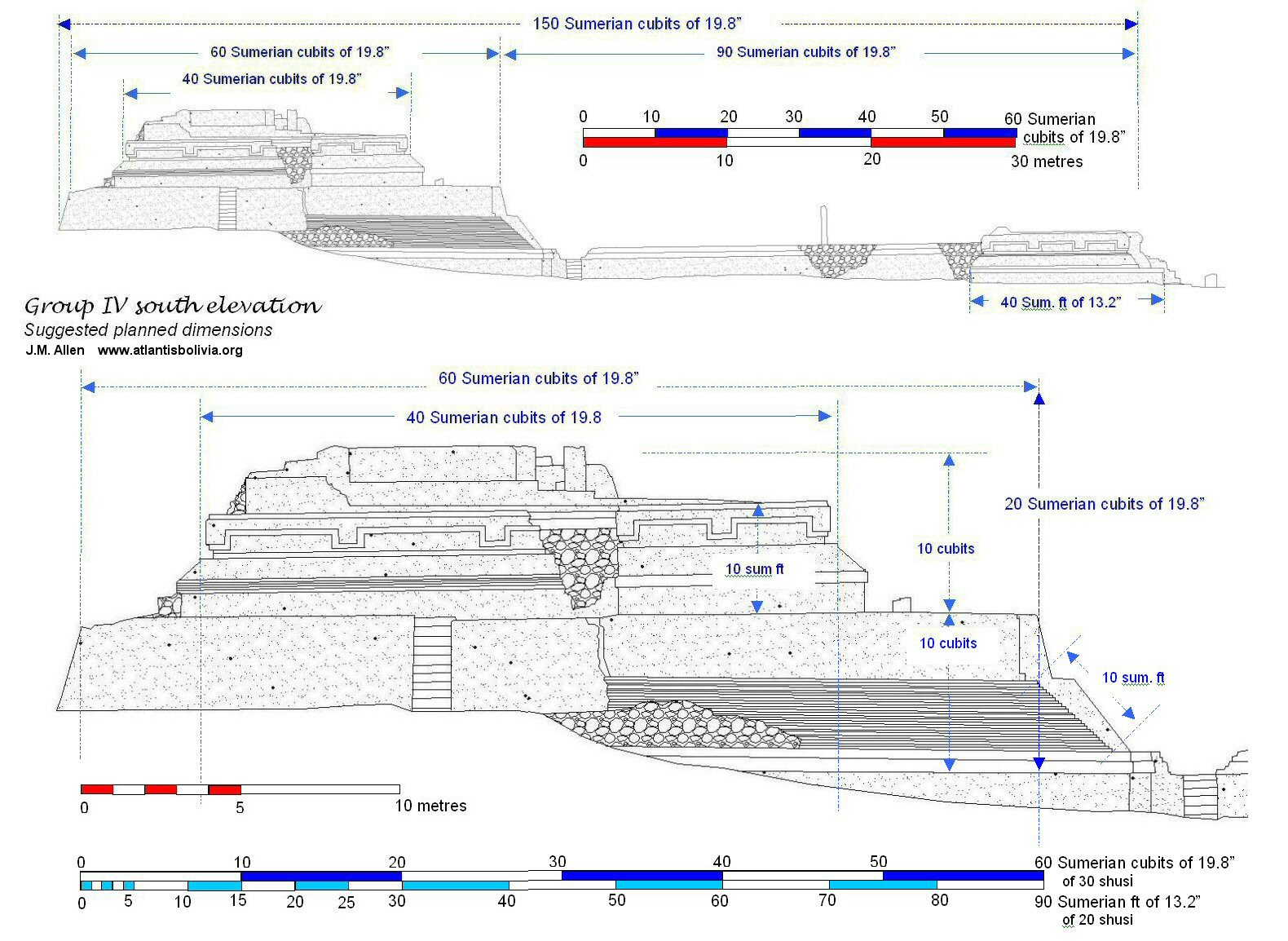above, the scaled measurements in Sumerian feet and cubits suggest a direct relationship between the height of the complex and its width.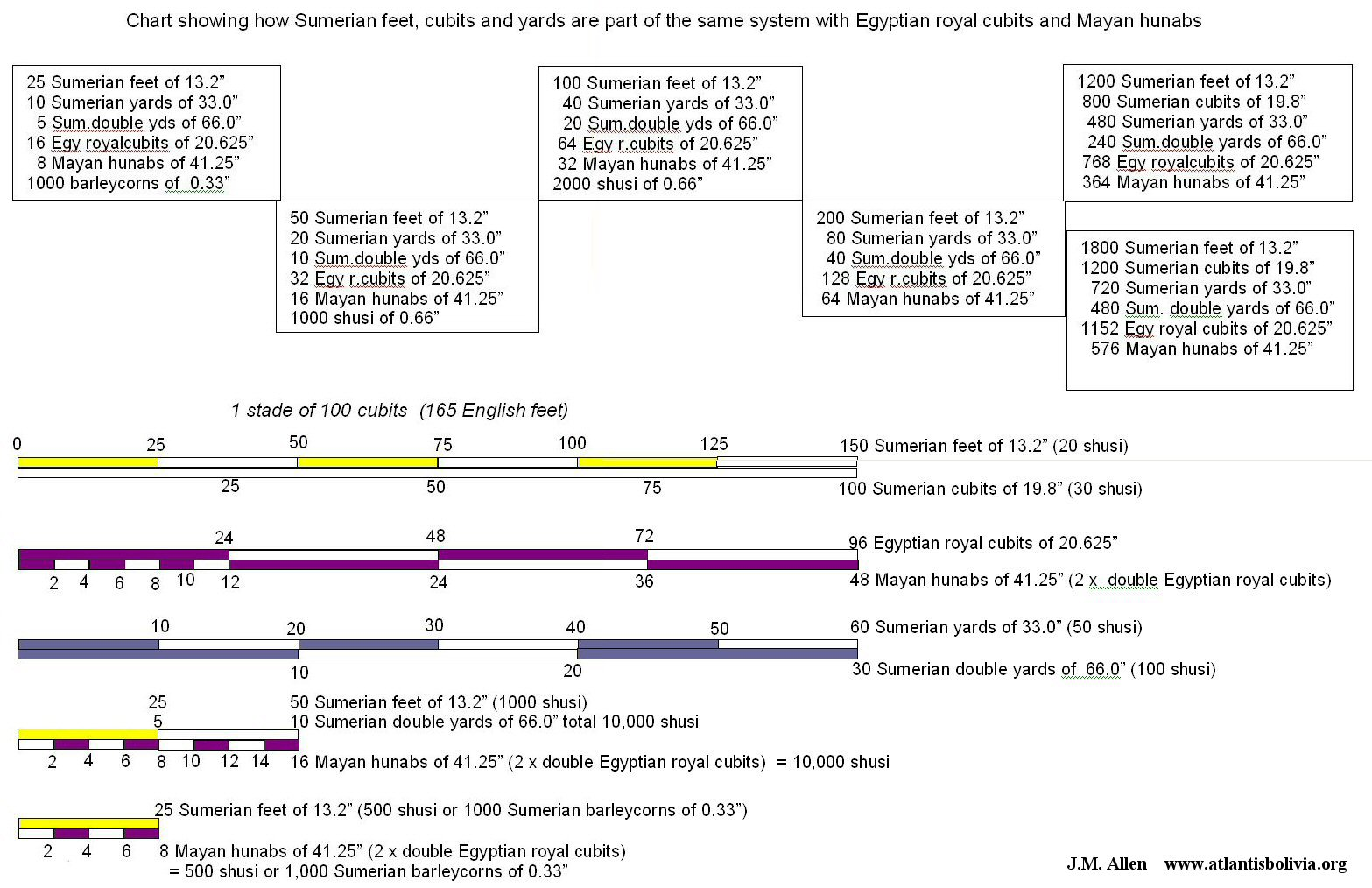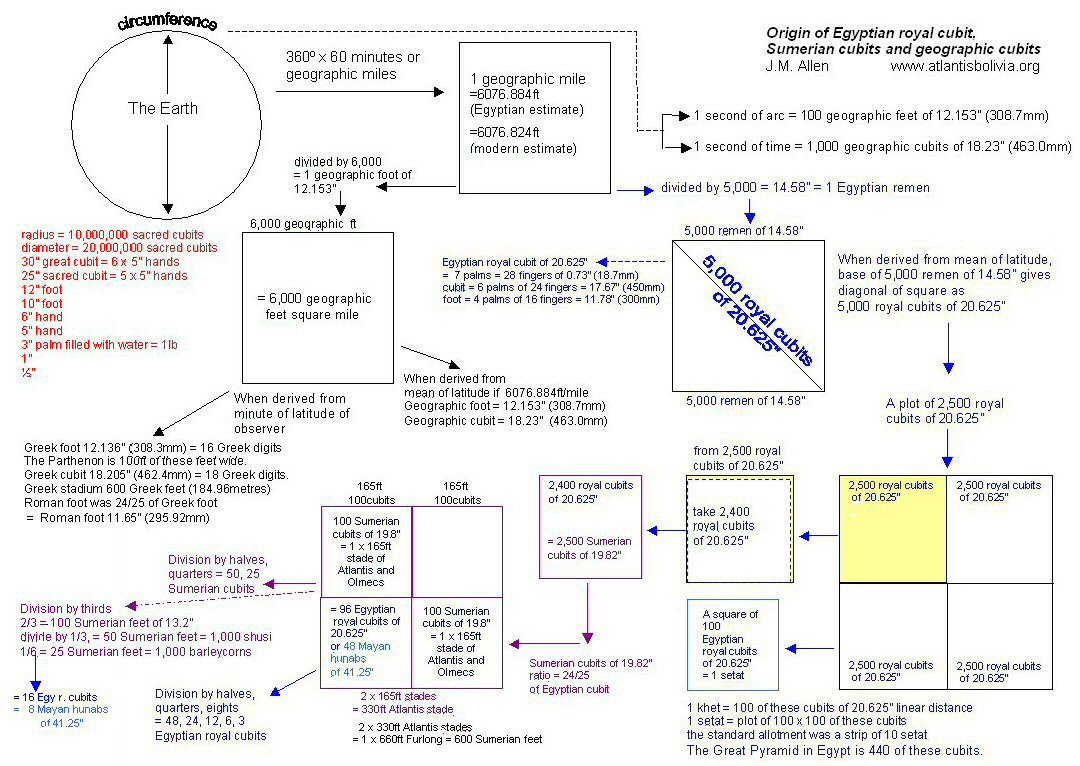Looking at the above chart we can see why Plato said the numbers 5 and 6 did "honour to the odd and even".

Taking the circumference of the world as 360º, each degree comprises 60 "geographic" or "nautical" miles. We can then choose to divide the geographic mile by 6,000 for geographic feet, or by 5,000 for Egyptian remen.

But first, we have four different ways to calculate the geographic mile. We could take the polar diameter x p and divide by 360 x 60. Or we could take the equatorial diameter x p divided by 360 x 60.

There again we could take a minute of latitude of where we lived and use that as our geographic mile which would vary depending on whether we lived in Greece, Athens or Babylonia, or alternatively, for a more Universal geographic mile we could take the mean figure for a minute of latitude and use that.

In the above chart, the Greeks to calculate the Greek mile or geographic mile used a 1/6000th part of a minute of latitude in Greece.

In Egypt on the other hand, they took a 5,000th part of a geographic mile based on the mean figure for a geographic mile and called this distance 1 x remen. From the base of a square of 5,000 remen, they obtained a diagonal which became 5,000 Egyptian Royal Cubits.

This was very useful for land surveying since in order to set out a square of 100 royal cubits, all they had to do was to set out a base line of 200 remen and intersect two arcs of 100 royal cubits on one side of the base line, and intersect 2 arcs of 100 royal cubits on the other side of the base line.

The Egyptian royal cubit comprised 28 fingers which was 7 palms, whereas 6 palms or 24 fingers made the regular Egyptian cubit and 4 palms or 16 fingers made the Egyptian foot.

If you set out a square whose sides were 2400 Egyptian royal cubits of 20.625", and then divided by 2500 you would then have units of Sumerian cubits of 19.8"

100 of these cubits made an "Atlantean" or "Olmec" stade which comprised 150 Sumerian feet, 60 Sumerian yards, or 30 Sumerian double-yards of 100 Sumerian shusi while if you divided it by 96 it became 96 Egyptian royal cubits or dividing by 48 gave Mayan hunabs so 1 x Mayan hunab was 2 x Egyptian royal cubits.

The differences arose depending on your preferred mathematical method, whether you divided by halves, quarters or eighths or by thirds while the Babylonians/Sumerians generally preferred 60's, the Egyptians 10's and the Mayans 20's ….

Tiwanaku calendar

Atlantis cubits between Altiplano canals

Teotihuacan measuring unit